•5星
5KB fangyango 2021-07-28 02:36:22
•5星
7.84MB yunlong3267 2021-07-23 16:49:56
•5星
38.87MB qq_42891281 2020-11-05 17:27:48
•5星
25KB weixin_54707168 2021-08-14 12:19:34
•5星
133KB weixin_43328149 2021-06-20 16:55:21
•5星
222KB qq_39397927 2021-06-26 21:02:31
•5星
220KB weixin_48465965 2021-08-07 15:58:07
•5星
11.24MB www_vane 2021-05-24 17:26:32
•5星
43KB qq_34093397 2021-01-04 10:45:38
•5星
116.59MB weixin_42818665 2021-05-15 21:21:36
• 求最大边权最小的生成树 c++

题目描述 Input Output Example ...一个任务的完成时间由最长的那条决定，而要找出最短的时间，那么就是计算最大边权最小生成树，也叫瓶颈树。 理解到这里以后，题目就相当容易了，考虑到K...

题目描述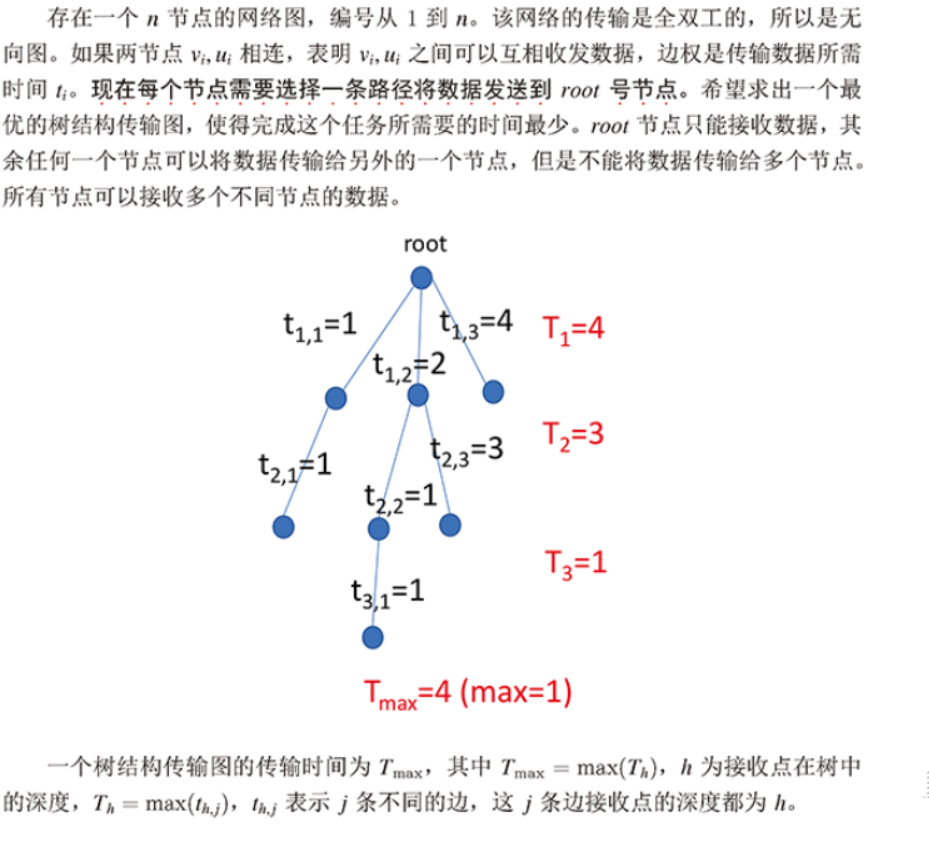Input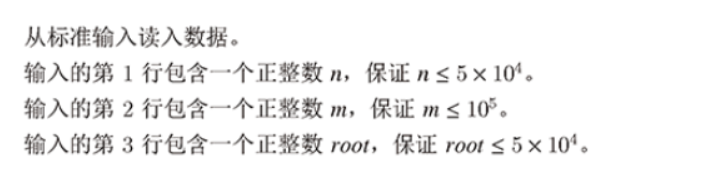Output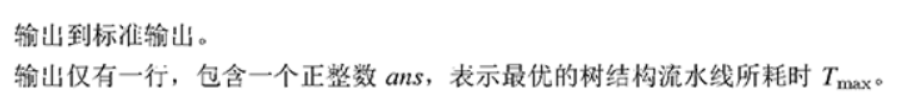Input

4
5
1
1 2 3
1 3 4
1 4 5
2 3 8
3 4 2

Output

4

解题思路

本题的题目看起来很复杂，可能要花很久才能理解题意，但是理解以后就很容易了。一个任务的完成时间由最长的那条边决定，而要找出最短的时间，那么就是计算最大边权最小的生成树，也叫瓶颈树。
理解到这里以后，题目就相当容易了，考虑到Kruskal算法得到的最小生成树本身就是按照边权由小到大生成的，其实就是一道最小生成树的题目。Kruskal算法便可以轻易求解（算法内容看上一篇文章）。

代码

#include<iostream>
#include<algorithm>
#include<cstring>
using namespace std;
const int maxn=50010;//点个数的最大值
const int maxm=100010;//边个树的最大值
struct Edge
{
int u,v,w;//出发点，到达点，权值
}Edges[maxm];
//int tot=0;//边的数目
bool cmp(Edge x,Edge y)
{
return x.w<y.w;
}
int par[maxn],rnk[maxn];
void init(int n) { for(int i=0;i<n;i++) par[i]=i, rnk[i]=1; }
int find(int x) { return par[x]==x?x:par[x]=find(par[x]); }
bool unite(int x,int y)
{
x=find(x),y=find(y);
if(x==y) return false;

if(rnk[x]>rnk[y]) swap(x,y);
par[x]=y;
rnk[x]+=rnk[y];
rnk[y]=rnk[x];
}
int main()
{

int n,m,root,weight;//点的个数和边的权值
cin>>n;//农田个数
init(n);//初始化并查集
cin>>m;//边的个数
cin>>root;//根节点的值
for(int i=0;i<m;i++)
{
cin>>Edges[i].u;
cin>>Edges[i].v;
cin>>Edges[i].w;
}
sort(Edges,Edges+m,cmp);
int start=0;//边集中选的边
int i;
for(i=0;i<n-1;i++)
{
while(find(Edges[start].u)==find(Edges[start].v))//按照权值由小到大的顺序找到第一个不成环的一条边
start++;
unite(Edges[start].u,Edges[start].v);
start++;
}
cout<<Edges[start-1].w<<endl;
return 0;
}

反思与体会

要学会读懂题目并理解题目含义，不要觉得题目很长就很难做，也许理解内容后会发现是一道很贱的题目。

展开全文weixin_43679657 2020-04-05 22:49:49
• 最小生成树——（性质）其最大边权为生成树中最大边权最小的——（例题）承包池塘的青蛙 最小生成树

最小生成树不仅可以得到最小的权值之和，其最大边权为生成树中最大边权最小的。*一道例题：1:承包池塘的青蛙 查看 提交 统计 提问 总时间限制: 1000ms 内存限制: 128000kB 描述 某池塘有N片荷叶, M只青蛙, 青蛙...

最小生成树不仅可以得到最小的权值之和，其最大边权为生成树中最大边权最小的。

*一道例题

1:承包池塘的青蛙
查看 提交 统计 提问
总时间限制: 1000ms 内存限制: 128000kB
描述
某池塘有N片荷叶, M只青蛙, 青蛙喜欢在荷叶之间跳来跳去.

给出青蛙们的最大可跳跃的距离Ci, 以及荷叶的坐标, 当忽略荷叶的直径.如果两片荷叶A和B间的距离小于某只青蛙F的最大可跳跃距离时, 可认为青蛙F可以从A跳到B, 也可以从B跳到A.

求能够从任意荷叶出发, 仅通过跳跃就能到达其它任意荷叶的青蛙个数.

输入
第一行一个整数, 表示M（2<=M<=500）
第二行为M个整数, 依次表示Ci（每个整数值在1—1000之间）
第三行为一个整数, 表示N（2<=N<=1000）
第四行至第N+3行, 每行两个数, 分布表示N个荷叶的坐标（横纵坐标均为整数, 范围为：-10000–10000）
输出
输出只有一行, 即所求满足条件的青蛙只数.
样例输入
4
100 200 300 400
6
0 0
100 0
100 200
-100 -100
-200 0
200 200
样例输出
3

疑问在于：为什么最小生成树的最长边是青蛙跳跃距离的下限？

首先，青蛙要到达所有节点，生成的图一定是经过所有点的。
存在无数个这样的图，而决定青蛙能否成功的只有这张图的最长边；
该图可能有环也可能无环，而有环图可以删掉某些边变为无环图，最长边只可能变小了或不变->最长边下线必定会在无环图（生成树）中找到；

确定了路线一定为生成树，接下来讨论为何是最小生成树。
我们用反证法：存在最小生成树，最长边为e；假设存在一个一般生成树，最长边eb且eb

#include <iostream>
#include <queue>
#include <list>
#include <stdio.h>
#include <vector>
#include <stdlib.h>
#include <string.h>
using namespace std;
class edge
{
public:
edge(int v1, int v2, int weigh)
{
_v1 = v1;
_v2 = v2;
_weigh = weigh;
}
int either()
{
return _v1;
}
int other(int v)
{
if(v == _v1) return _v2;
return _v1;
}
int weigh()
{
return _weigh;
}
private:
int _v1;
int _v2;
int _weigh;
};
struct cmp
{
bool operator()(edge a, edge b)
{
return a.weigh() > b.weigh();
}
};
class mst
{
public:
mst(int v)
{
_v = v;
_marked = new bool[v];
memset(_marked, false, sizeof(_marked));
_max = 0;
}
void insert(edge e)
{
int v1 = e.either();
int v2 = e.other(v1);
}
void build(int s)
{
visit(s);
while(!q.empty()){
edge cur = q.top();
q.pop();
int v1 = cur.either();
int v2 = cur.other(v1);
if(_marked[v1] && _marked[v2]) continue;
if (cur.weigh() > _max) _max = cur.weigh();
if(!_marked[v1]) visit(v1);
else if(!_marked[v2]) visit(v2);
}
}
void visit(int p)
{
_marked[p] = true;
int len = nei.size();
for(int i = 0; i < len; i++){
q.push(nei[i]);
}
}
int max()
{
return _max;
}
private:
priority_queue <edge, vector<edge>, cmp> q;
bool* _marked;
int _v;
int _max;
};
int main()
{
int m;
cin >> m;
int frog;
for(int i = 0; i < m; i++){
cin >> frog[i];
}
int n;
cin >> n;
int co;
for(int i = 0; i < n; i++){
cin >> co[i] >> co[i];
}
mst pond(n);
int numOfEdge = (n * (n - 1)) / 2;
for(int j = 0; j < n - 1; j++){
for(int k = j + 1; k < n; k++){
int x1 = co[j];
int y1 = co[j];
int x2 = co[k];
int y2 = co[k];
int weigh2 = (x1 - x2) * (x1 - x2) + (y1 - y2) * (y1 - y2);
edge cur(j, k, weigh2);
pond.insert(cur);
}
}
pond.build(0);
int max = pond.max();
int count = 0;
for(int i = 0; i < m; i++){
if(frog[i] * frog[i] >= max) count++;
}
cout << count << endl;
return 0;
}
展开全文wuzhihao1995 2016-03-31 00:50:19
•5星
2KB weixin_42681774 2021-10-02 07:54:00
• 最小生成树：在一个给定的无向G（V，E）中求一颗树T，使这棵树拥有G中的所有顶点，且所有都来自于G中的，并且满足整颗树的边权之和最小。 1.2 性质 1 最小生成树是树，因此其数等于其顶点树减一，且树...

1 概念

1.1 定义

最小生成树：在一个给定的无向图G（V，E）中求一颗树T，使这棵树拥有图G中的所有顶点，且所有边都来自于图G中的边，并且满足整颗树的边权之和最小。

1.2 性质

• 1 最小生成树是树，因此其边数等于其顶点树减一，且树内一定不会有环；
• 2 对于给定的图G(V,E),其最小生成树可以不唯一，但其边权之和一定唯一；
• 3 由于最小生成树是无向图上生成的，因此其根结点可以是这颗树上的任意结点。

2 求解最小生成树

2.1 prim算法（普里姆算法）

2.1.1 算法思想

• 对于图G（V，E）设置集合S来存放已被访问的顶点，然后执行n次下面的两个步骤：
• 1 每次从集合V-S中选择与集合S最近的一个顶点（记为u），访问u并将其加入集合S，同时把这条离集合S最近的边加入最小生成树中，
• 2 令顶点u作为集合S和集合V-S连接的接口，优化从u能到达的未访问的顶点v与集合S的最短距离

2.1.2 模版伪代码

//图G一般设置为全局变量；数组d为顶点与集合S的最短距离
Prim(G, d[]){
初始化;
for (循环n次)
{
u = 使d[u]最小的海未被访问的顶点标号;
记u已被访问;
for (从u出发能到达的所有顶点v)
{
if(v未被访问&&以u为中介点使得v与集合S的最短距离d[v]更优){
将G[u][v]赋值给v与集合S的最短距离d[v];
}
}
}
}

2.1.3 代码

2.1.3.1 邻接矩阵
const int MAXV = 1000;
const int INF = 0x3fffffff;
int G[MAXV][MAXV];//图
int n;//顶点个数
bool vis[MAXV] = {false};//记录顶点是否被访问
int d[MAXV];//顶点与集合S的最短距离

int prim(){//默认0号为初始点，函数返回最小生成树的边权之和
fill(d, d + MAXV, INF);
d = 0;//只有0号顶点到集合S的距离为0，其余为INF
int ans = 0;//存放最小生成树的边权之和
for (int i = 0; i < n; ++i)
{
int u = -1, min = INF;
for (int j = 0; j < n; ++j)
{
if(vis[j] == false && d[j] < min){
u = j;
min = d[j];
}
}
//找不到小于INF的d[u]，则剩下的顶点和集合S不连通
if(u == -1) return -1;
vis[u] = true;
ans += d[u];//将与集合S距离最小的边加入最小生成树

for (int v = 0; v < n; ++v)
{
//v未访问 && u能到达v && 以u为中介点可以是v离集合S更近
if(vis[v] == false && G[u][v] != INF && G[u][v] < d[v]){
d[v] = G[u][v];
}
}
}

return ans;
}

2.1.3.2 邻接表
struct node
{
int v;
int dis;
};

const int MAXV = 1000;
const int INF = 0x3fffffff;
vector<node> G[MAXV];//图
int n;//顶点个数
bool vis[MAXV] = {false};//记录顶点是否被访问
int d[MAXV];//顶点与集合S的最短距离

int prim(){//默认0号为初始点，函数返回最小生成树的边权之和
fill(d, d + MAXV, INF);
d = 0;//只有0号顶点到集合S的距离为0，其余为INF
int ans = 0;//存放最小生成树的边权之和
for (int i = 0; i < n; ++i)
{
int u = -1, min = INF;
for (int j = 0; j < n; ++j)
{
if(vis[j] == false && d[j] < min){
u = j;
min = d[j];
}
}
//找不到小于INF的d[u]，则剩下的顶点和集合S不连通
if(u == -1) return -1;
vis[u] = true;
ans += d[u];//将与集合S距离最小的边加入最小生成树

for (int j = 0; j < G[u].size(); ++j)
{
int dis = G[u][j].dis;
int v = G[u][j].v;
//v未访问  && 以u为中介点可以是v离集合S更近
if(vis[v] == false && dis < d[v]){
d[v] = dis;
}
}
}

return ans;
}

2.1.4 注意点

• 上面写法的算法时间复杂度为O(V2)；
• 邻接表的写法可以通过堆优化，将时间复杂度降到O(VlogV+E)
• 尽量在顶点较少边较多的情况下（稠密图），使用prim算法

2.1.5 示例

输入：
6 10 //6个顶点，10条边。以下10行为10条边
0 1 4 //边0->1和1->0的边权为4，下同
0 4 1
0 5 2
1 2 6
1 5 3
2 3 6
2 5 5
3 4 4
3 5 5
4 5 3
输出：
15 //最小生成树边权

#include <cstdio>
#include <algorithm>
#include <vector>

using std::vector;
using std::fill;

struct node
{
int v;
int dis;
node(int _v, int _dis): v(_v), dis(_dis){}
};

const int MAXV = 1000;
const int INF = 0x3fffffff;
vector<node> G[MAXV];//图
int n;//顶点个数
bool vis[MAXV] = {false};//记录顶点是否被访问
int d[MAXV];//顶点与集合S的最短距离

int prim(){//默认0号为初始点，函数返回最小生成树的边权之和
fill(d, d + MAXV, INF);
d = 0;//只有0号顶点到集合S的距离为0，其余为INF
int ans = 0;//存放最小生成树的边权之和
for (int i = 0; i < n; ++i)
{
int u = -1, min = INF;
for (int j = 0; j < n; ++j)
{
if(vis[j] == false && d[j] < min){
u = j;
min = d[j];
}
}
//找不到小于INF的d[u]，则剩下的顶点和集合S不连通
if(u == -1) return -1;
vis[u] = true;
ans += d[u];//将与集合S距离最小的边加入最小生成树

for (int j = 0; j < G[u].size(); ++j)
{
int dis = G[u][j].dis;
int v = G[u][j].v;
//v未访问  && 以u为中介点可以是v离集合S更近
if(vis[v] == false && dis < d[v]){
d[v] = dis;
}
}
}
return ans;
}

int main(int argc, char const *argv[])
{
int m, u, v, w;
scanf("%d%d", &n, &m);
for (int i = 0; i < m; ++i)
{
scanf("%d%d%d", &u, &v, &w);
G[u].push_back(node(v,w));
G[v].push_back(node(u,w));
}

int ans = prim();
printf("%d\n", ans);
return 0;
}
/*
6 10
0 1 4
0 4 1
0 5 2
1 2 6
1 5 3
2 3 6
2 5 5
3 4 4
3 5 5
4 5 3
*/

2.2 kruskal算法（克鲁斯卡尔算法）

2.2.1基本思想

• 在初始状态时，隐去图中所有边，这样图中每个顶点都自成一个连通块，。之后执行下面的步骤：
• 1 对所有边按边权按从小到大排序；
• 2 按边权从小到大测试所有边，如果当前测试边所连接的两个顶点不在同一个连通块中，则把这条测试边加入当前最小生成树中；否则，将边舍弃；
• 3 执行步骤2， 直到最小生成树中的边数等于顶点树减1或是测试完所有边时结束。而当结束时，如果最小生成树的边数小于总顶点树减1，说明该图不连通。
• 简单说：每次选择图中最小边权的边，如果边两端的顶点在不同连通块中，则把这条边加入最小生成树中，

2.2.2 模版伪代码

• 1 边的定义
因为需要判断边的两个断点是否在不同的连通块中，因此边的两个端点编号一定需要；算法涉及边权，因此边权也需要。
struct edge
{
int u, v;//边的两个端点编号
int cost;//边权
};

• 2 排序函数
让数组E按边权从小到大排序
bool cmp(edge a, edge b){
return a.cost < b.cost;
}

• 3 伪代码
int krukal(){
令最小生成树的边权之和为ans、最小生成树的当前边数为Num_Edge;
将所有边按边权大小排序;
for(从小到大枚举所有的边){
if(当测试的两个端点在不同连通块中){
将测试边加入最小生成树中;
ans += 测试边的边权;
最小生成树的当前边权Num_Edge加1;
当边数Num_Edge等于顶点数减1时结束循环;
}
}
return ans;
}

2.2.3 实现代码

const int MAXV = 120;//最多顶点数
const int MAXE = 1000;//最多边数
int father[MAXV];//并查集数组

struct edge
{
int u, v;//边的两个端点编号
int cost;//边权
}E[MAXE];

int findFather(int x){
int a = x;
while(x != father[x]){
x = father[x];
}

while(a != father[a]){
int z = a;
a = father[a];
father[z] = x;
}

return x;
}

bool cmp(edge a, edge b){
return a.cost < b.cost;
}

int krukal(int n, int m){
int ans = 0, Num_Edge = 0;//ans:所求边权之和；Num_Edge：当前生成树的边数
for (int i = 1; i <= n; ++i)//假设顶点范围为1~n
{
father[i] = i;//并查集初始化
}
sort(E, E + m, cmp);//所有边权从小到大排序

for (int i = 0; i < m; ++i)//枚举所有边
{
int faU = findFather(E[i].u); //查询测试边的两个端点所在集合的根结点
int faV = findFather(E[i].v);
if(faU != faV){//如果不在一个集合中
father[faU] = faV;//合并集合（把测试边加入最小生成树）
ans += E[i].cost;//边权之和加入测试边的边权
Num_Edge++;//当前生成树边数加1
if(Num_Edge == n - 1){//边数等于顶点树-1时，结束算法
break;
}
}
}
if(Num_Edge != n - 1){//无法连接时，返回-1
return -1;
}else{//返回最小生成树的边权之和
return ans;
}
}

2.2.4 注意

*时间复杂度主要在于对边进行排序，时间复杂度为O(ElogE)

• 适合顶点较多，边数较少的情况（稀疏图）

2.2.5 示例

输入：
6 10 //6个顶点、10条边，下面跟着10条无向边
0 1 4 //0号顶点与1号顶点的无向边的边权为4
0 4 1
0 5 2
1 2 1
1 5 3
2 3 6
2 5 5
3 4 5
3 5 4
4 5 3
输出：
11 //最小生成树的边权之和

#include <cstdio>
#include <algorithm>

using std::sort;

const int MAXV = 110;
const int MAXE = 10000;
int father[MAXV];

struct edge
{
int u, v;
int cost;
}E[MAXE];

bool cmp(edge a, edge b){
return a.cost < b.cost;
}

int findFather(int x){
int a = x;
while(x != father[x]){
x = father[x];
}

while(a != father[a]){
int z = a;
a = father[a];
father[z] = x;
}

return x;
}

int kruskal(int n, int m){
int ans = 0, Num_Edge = 0;
for (int i = 0; i < n; ++i)
{
father[i] = i;
}

sort(E, E + m, cmp);
for (int i = 0; i < m; ++i)
{
int faU = findFather(E[i].u);
int faV = findFather(E[i].v);
if(faU != faV){
father[faU] = faV;
ans += E[i].cost;
Num_Edge++;
if(Num_Edge == n - 1){
break;
}
}
}
if(Num_Edge != n - 1){
return -1;
}else {
return ans;
}
}

int main(int argc, char const *argv[])
{
int n, m;
scanf("%d%d", &n, &m);
for (int i = 0; i < m; ++i)
{
scanf("%d%d%d", &E[i].u, &E[i].v, &E[i].cost);
}
int ans = kruskal(n, m);
printf("%d\n", ans);
return 0;
}

/*
6 10
0 1 4
0 4 1
0 5 2
1 2 1
1 5 3
2 3 6
2 5 5
3 4 5
3 5 4
4 5 3
*/

展开全文qq_33375598 2020-02-21 16:47:22
• 1KB jemiesama 2016-11-25 12:32:59
• 2KB mujiang770419151 2010-06-26 16:13:02
• 1014B qq_38243583 2017-11-12 14:22:54
• 图的应用——最小生成树 最小生成树 Prim KrusKal 数据结构

最小生成树最小生成树构造最小生成树的准则贪心算法(Greedy Algorithm)Prim（普里姆）算法算法思想 —— 归并顶点算法设计KrusKal（克鲁斯卡尔）算法算法思想 —— 归并算法设计Prim和KrusKal比较 最小生成树 ...

最小生成树

• 生成树（极小连通子图）：含有图中全部n个顶点，但只有n-1条边。并且n-1条边不能构成回路。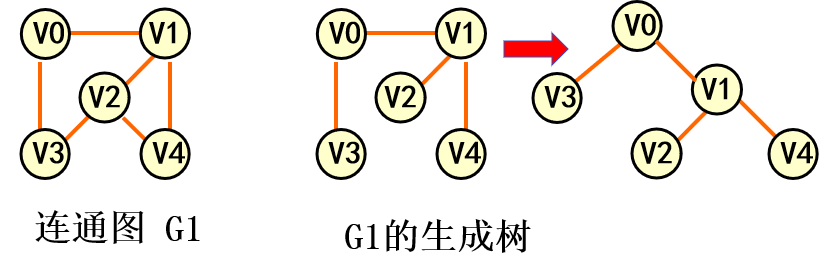• 生成森林：非连通图每个连通分量的生成树一起组成非连通图的生成森林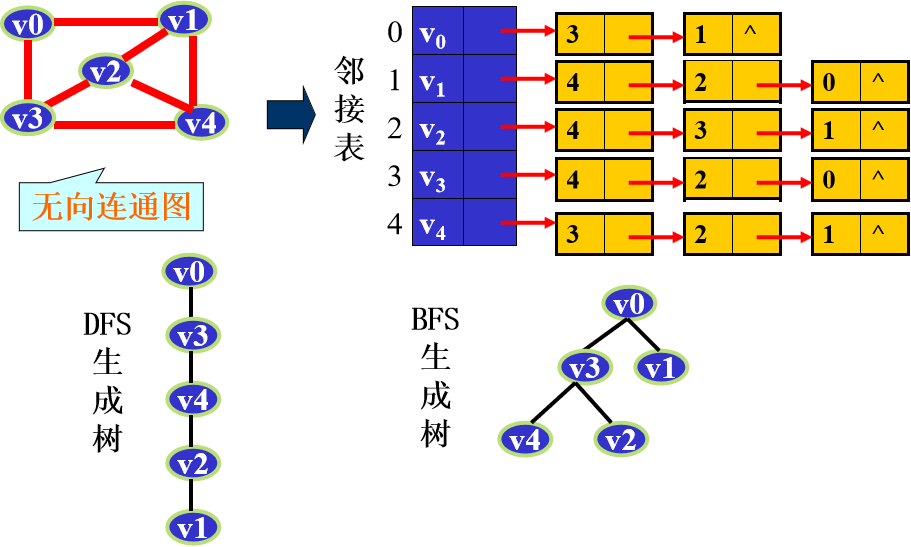求最小生成树

• 使用不同的遍历图的方法，可以得到不同的生成树
• 从不同的顶点出发，也可能得到不同的生成树。
• 按照生成树的定义，n 个顶点的连通网络的生成树有 n 个顶点、n-1 条边。

在网的多个生成树中，寻找一个各边权值之和最小的生成树

构造最小生成树的准则

• 必须只使用该网中的边来构造最小生成树；
• 必须使用且仅使用n-1条边来联结网络中的n个顶点
• 不能使用产生回路的边

贪心算法(Greedy Algorithm)

• 算法原理：以当前情况为基础作最优选择，而不考虑各种可能的整体情况，所以贪心法不要回溯。
• 算法优点：因为省去了为寻找解而穷尽所有可能所必须耗费的大量时间，因此算法效率高。

贪婪算法的精神就是“只顾如何获得眼前最大的利益”,有时不一定是最优解。

Prim（普里姆）算法

算法思想 —— 归并顶点
• 在图中任取一个顶点K作为开始点令U={k}，W=V-U，其中V为图中所有顶点集
• 在U中选取一个顶点，W中选取另一个顶点，使二者对应的边是最短的一条。将该边作为最小生成树的边保存起来，并将该边顶点全部加入U集合中，并从W中删去这些顶点。
• 重新调整U中顶点到W中顶点的距离， 使之保持最小，再重复此过程，直到W为空集止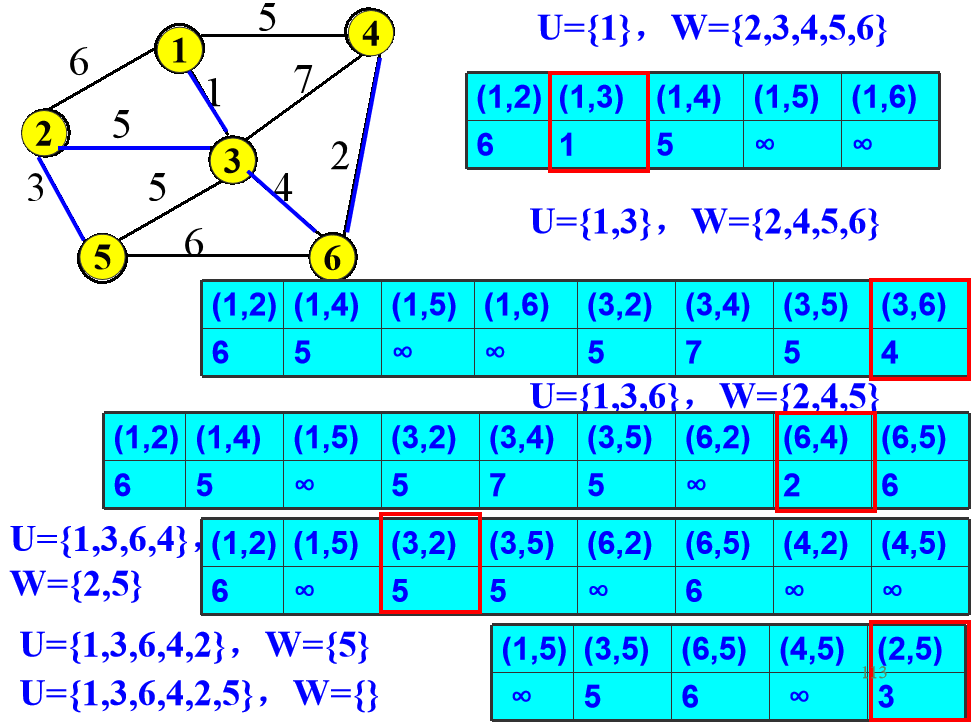算法设计
• 在算法中需要设置一个辅助数组，对当前V－U集中的每个顶点，记录和顶点集U中顶点相连接的代价最小的边
struct {
VRType lowcost;  // 边的权值
} closedge[MAX_VERTEX_NUM];

• lowcost[i]:表示以i为终点的边的最小权值,当lowcost[i]=0说明以i为终点的边的最小权值=0,也就是表示i点加入了MST
void MiniSpanTree_P(MGraph G, VertexType u){
// 用普利姆算法从顶点u出发构造网G的最小生成树
k = LocateVex_MG(G, u);
for(j = 0; j < G.vexnum; ++j)  // 辅助数组初始化
if(j != k){
}
closedge[k].lowcost = 0;  // 初始, U = {u}
for(i = 0; i > G.vexnum; i ++){
// //继续向生成树上添加顶点
mincost = INF;  // 找权值最小的顶点
for(m = 0; m < G.vexnum; ++m)
if(mincose > closedge[m].lowcost && closedge[m].lowcost != 0){
mincose = closedge[m].lowcost;
k = m;
} // 求出加入生成树的下一个顶点(k)
if(closedge[k].lowcost != 0)
//输出生成树上一条边
cout << closedge[k].adjvex << G.vexs[k] << closedge[k].lowcost;
closedge[k].lowcost = 0; // 第k顶点并入U集
for(j = 0; j < G.vexnum; j++)
// 修改其它顶点的最小边
}
}
}

KrusKal（克鲁斯卡尔）算法

算法思想 —— 归并边
• 将图中所有边按权值递增顺序排列；
• 依次选定取权值较小的边，但要求后面选取的边不能与前面选取的边构成回路，若构成回路，则放弃该条边，再去选后面权值较大的边，n个顶点的图中，选够n-1条边即可。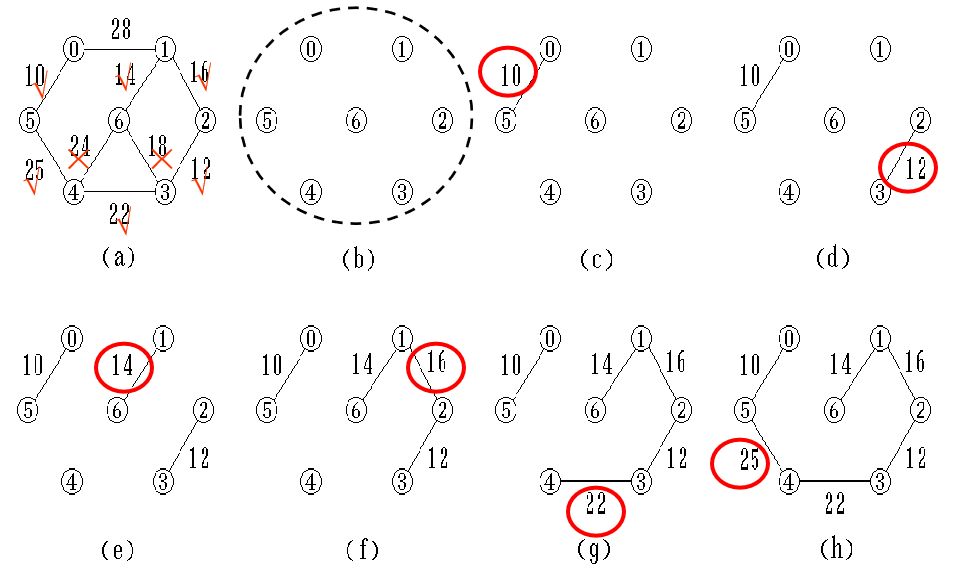算法设计

构造非连通图 ST=( V,{ } );
k = i = 0; // k 计选中的边数
while (k<n-1) {
++i;
检查边集 E 中第 i 条权值最小的边(u,v);
若(u,v)加入ST后不使ST中产生回路，
则 输出边(u,v); 且 k++;

}

typedef struct {
// 增加边结构定义
int beginvex, endvex;  // 边的起点、终点
VRType cost;  // 边的权值
} edgetype;

edgetype edges[MAX_VERTEX_NUM];

void MiniSpanTree_Kruskal(ALGraph &G){
int parents[MAX_VERTEX_NUM];
cin >> G.vexnum >> G.arcnum;  // 顶点数、弧数
for(i = 0; i < G.vexnum; i++){
// 建立顶点表
G.vertices[i].data = new char;
cin >> G.vertices[i].data;  // 读入顶点信息并初始化
G.vertices[i].firstarc = NULL;
}
for(k = 0; k < G.arcnum; k++){
// 建立边表
v1 = new char;
v2 = new char;
cin >> v1 >> v2 >> w;
i = LocateVex_ALG(G, v1);
j = LocateVex_ALG(G, v2);
edges[k].beginvex = i;
edges[k].endvex = j;
edges[k].cost = w;
p = new ArcNode;
p->info = NULL;
p->nextarc = G.vertices[i].firstarc;
G.vertices[i].firstarc = p;
}
Sort(G, edges);  // 按权值大小，对边进行排序
for(i = 0; i < G.vexnum; i++)
parents[i] = 0;
for(i = 0; i < G.arcnum; i++){
bnf = Find(parents, edges[i].beginvex);  // 查找边头分量
edf = Find(parents, edges[i].endvex);  // 查找边尾分量
if(bnf != edf){
parents[bnf] = edf;
cout << edges[i].beginvex << edges[i].endvex;
cout << " " << edges[i].cost << endl;
}
}
}

int Find(int parents[], int f){
// 查找函数
while(parents[f] > 0) f = parents[f];
return f;
}

Prim和KrusKal比较

算法名PrimKrusKal
时间复杂度O(n^2)O(eloge)
适应范围稠密图稀疏图
展开全文qq_29339467 2019-12-30 14:25:02
• 505KB qq_33874363 2016-06-03 23:50:15
• 加权无向图与最小生成树（Prim算法和Kruskal算法） 数据结构 最小生成树 加权无向图 Prim算法 Kruskal算法

sc179 2020-12-07 16:54:27
• 2.6MB ziyanglanyue 2016-04-10 13:56:31
• 最小生成树详解(模板 + 例题) 最小生成树 MST

qq_43619271 2020-10-15 16:04:26
• python中的图结构——最小生成树 python 最小生成树

nanashi_F 2019-06-16 11:34:16
• 克鲁斯卡尔（Kruskal）算法之加权连通图的最小生成树问题 克鲁斯卡尔算法 Kruskal算法 最小生成树问题

qq_16645099 2020-03-31 22:23:54
• 87KB hgyyj 2011-05-24 23:02:53
• 最小生成树 加权无向图 加权无向图 最小生成树

gaoruowen1 2018-11-06 13:45:11
• 最小生成树设G=（V，E）是无向图联通带权图，即一个网络。E中每条边（v，w）的权为c[v][w]。如果G的一个子... 最小生成树4星
1KB qinchaohan 2009-03-11 15:02:43
• 最小生成树（带权无向图） 最小生成树 Prim算法

jyy555555 2018-04-14 11:19:05
• ITmincherry 2020-05-17 00:54:21
• 求的带权图最小生成树的Prim算法和Kruskal算法 Prim Kruskal 最小生成树

xiaoduan_ 2018-08-02 15:16:08
• 图的最小生成树（Prim算法） 算法

qq_41891805 2020-04-03 20:46:07
• softwareX4 2019-08-09 12:45:56
• [图]python实现图的遍历、最小生成树、最短路径 图遍历 最小生成树 最短路径 Dijkstra算法 Prime算法

woniu201411 2018-11-13 21:40:50
• 最小生成树（图解普里姆算法、图解克鲁斯卡尔算法） 算法 java 最小生成树 普里姆算法 克鲁斯卡尔算法

zhuxian1277 2021-02-04 20:17:17
• 最小生成树的三种算法及图的相关知识 最小生成树 生成树 Prim Kruskal

lxt_Lucia 2018-06-01 21:18:43
• 算法快学笔记（十四）：图的最小生成树算法 图算法 最小生成树 Prim Kruskal Python

sun7545526 2019-10-24 15:27:51
• 最小生成树问题的两种算法 最小生成树 prim kruskal java

GD_ONE 2020-02-20 01:04:06
• Python实现最小生成树（Prim算法） 最小生成树 Prim

qq_38038143 2019-04-27 11:04:06
• 数据结构之图--最小生成树（普里姆（Prim）算法） 最小生成树 Prim算法 普里姆算法

qq_31820761 2018-06-17 16:03:05
• 最小生成树详细讲解（Prime算法+Kruskalsuanfa） 最小生成树

qq_41181772 2019-04-17 17:53:48...